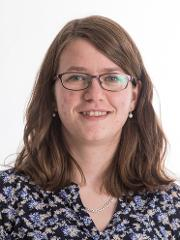autumn 2018

## MAT-2201 Numerical Methods - 10 stp

Sist endret: 25.02.2019

## The course is administrated by

Faculty of Science and Technology

## Studiested

Tromsø |

Applicants from Nordic countries: 1 June for the autumn semester and 1 December for the spring semester. Applicants from outside the Nordic countries: 1 October for the spring semester and 15 April for the autumn semester.

## Type of course

The course is mandatory i the study program Mathematics and Finance - master, and it's included in the study programs Mathematics and Statistics - bachelor and Applied Physics and Mathematics - master (5-years). It may also be taken independent of study program.

Applicants from Nordic countries:
Generell studiekompetanse og følgende spesielle opptakskrav:
Matematikk R1 + R2 og i tillegg enten:

• Fysikk 1 + 2 eller
• Kjemi 1+ 2 eller
• Biologi 1 + 2 eller
• Informasjonsteknologi 1 +2 eller
• Geofag 1 + 2 eller
• Teknologi og forskningslære 1 + 2

International applicants: Higher Education Entrance Qualification and certified language requirements in English. It is a requirement that students have  some prior knowledge of biology and ecology, chemistry and mathematics (Participants must have taken introductory level university courses, and achieved pass grades, in these subjects).

A list of the requirements for the Higher Education Entrance Qualification in Norway can be found on the Norwegian Agency for Quality Assurance in Education website - nokut.no

Recommended prerequsites is MAT-1003 Calkulus 3 and MAT-1004 Linear algebra or equal.

Application code: 9336 (Nordic applicants).

## Course contents

This course gives an introduction to basic concepts and issues of numerical computation. The topics treated include: Binary representation and floating point numbers, round-off errors, conditioning, rates of convergence, truncation and discretization errors, best approximation, numerical stability, and complexity analysis. Selected methods will be covered for some of these classes of problems: Linear systems of equations, nonlinear equations, overdetermined linear systems, numerical differentiation and integration, and numerical solution of differential equations.

## Recommended prerequisites

MAT-1002 Calculus 2, MAT-1004 Linear algebra

## Objective of the course

After the course the student should:

• Be able to analyze methods for numerical calculations with respect to errors and complexity
• Have mathematical understanding for the methods they apply
• Know the main features in IEEE-standards for binary number representation
• Be able to use iterative methods, like the Jacobi-method for systems of linear equations, and Newtons method for non-linear equations, and be able to describe convergence properties.
• Be able to describe Gaussian elimination and LU factorization, and know QR factorization, and how this is used to find least squares solutions.
• Know the problem of polynomial interpolation, how to solve it, and how to prove unqueness. They should be able to use Chebychev polynomials as tools.
• Use Taylor’s theorem to find errors of discretization when calculating dericatives and finite difference.
• Know simple methods for numerical calculation of integrals, such as the Trapezoid method and Simpson’s formula, and general results about global errors, when local errors are known.
• Know the simplest algorithms for stepwise numerical solution of initial value problems for systems of first order differential equations, and know how to reformulate a higher order differential equation to such a system.

## Language of instruction

The language of instruction and the syllabus is English. Examination questions will be given in English, but may be answered either in English or a Scandinavian language.

Lectures: 40 h
Coursework: 30 h

## Assessment

Written final exam of 4 hours duration, counting 100 %.

Postponed examination: Students with valid grounds for absence will be offered a postponed examination early in the following semester.

For further details see:
- Utfyllende bestemmelser for eksamener ved Fakultet for naturvitenskap og teknologi (only in Norwegian)
- Regulations for examinations at the University of Tromsø

Coursework requirements
A passing grade is required on the mandatory homework sets for permission to take the exam.

## Date for examination

Written test 05.12.2018

The date for the exam can be changed. The final date will be announced in the StudentWeb early in May and early in November.

## Course overlap

MA-224 Numerical calculations 10 stp
FYS-2011 Numerical simulations 10 stp

Curriculum for MAT-2201 Numerical Methods, autumn 2018
UiT The Arctic University of Norway, Department of Mathematics and Statistics

Textbook: T. Sauer: Numerical Analysis. 2nd edition. Pearson:
US edition (alternative 1):
ISBN-13: 978-0321783677
ISBN-10: 0321783670

or International edition (alternative 2):
ISBN13: 9781292023588

or new version (the one for sale in Akademika)
T. Sauer: Numerical Analysis. 3rd edition. Pearson:
ISBN-13: 978-0-13-469645-4
ISBN-10: 0-13-469645-X

Ch. 0. Fundamentals

Ch. 1. Solving equations
1.1 The bisection method
1.2 Fixed-point iteration
1.3 Limits of accuracy
1.4 Newton's method

Ch. 2. Systems of equations
2.1 Gaussian elimination
2.2 The LU factorization
2.3 Sources of error
2.4 The PA=LU factorization
2.5 Iterative methods

Ch. 3. Interpolation
3.1 Data and interpolating functions
3.2 Interpolation error

Ch. 4. Least squares
4.1 Least squares and the normal equations
4.2 A survey of models
4.3. QR factorization

Ch. 5. Numerical differentiation and integration
5.1 Numerical Differentiation
5.2 Newton-Cotes formulas (except 5.2.4 open Newton-Cotes methods)

Ch. 6. Ordinary differential equations
6.1 Initial value problem
6.2 Analysis of IVP solvers
6.3 Systems of Ordinary Differential Equations (except 6.3.2 and 6.3.3)
6.4 Runge-Kutta Methods (except 6.4.2 and 6.4.3)

Ch. 7. Boundary value problems
7.1 Shooting Method
7.2 Finite difference methods

Appendix AForhåndsvis dokumentet. Matrix Algebra

Compendium "p-Norm of vectors and matricesForhåndsvis dokumentet" is also a part of the syllabus

Appendix BForhåndsvis dokumentet (for those who use MATLAB): Introduction to MATLAB

###### Kontakt### Fredriksen, Hege-Beate

Postdoktor / Modellering av komplekse systemer
hege-beate.fredriksen@uit.no